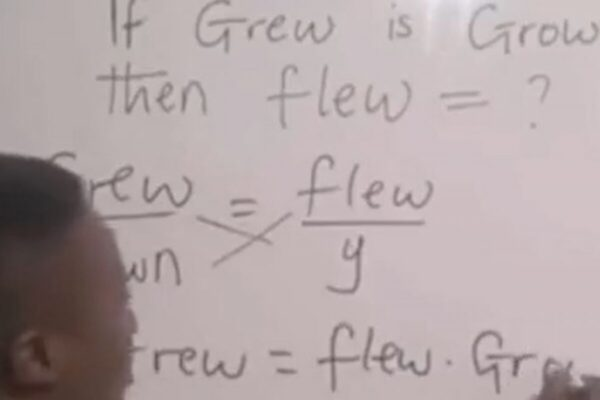Viral, Dec 15: A video of a man mathematically deriving the past participle of the word ‘flew‘ has created a buzz on social media. The man turned English grammar into a mathematical equation and netizens can’t stop laughing.

The video was posted on Twitter by former finance secretary Dr Arvind Mayaram. “Wicked Wit! When the English Teacher is on vacation and the Principal asks the Maths Teacher to take the English class!” Mayaram wrote in the caption.

In the video, the man can be seen writing “If grew is grown, then flew =?” on a blackboard. “Look at what a mathematician will do“.

He then uses the variable ‘y‘ to represent the past participle of flew and turns the four quantities into a mathematical equation by cross multiplying.

The equation “y X Grew = flew X GREW” is derived. The man goes on to solve the equation to find the solution that “y = flown“.